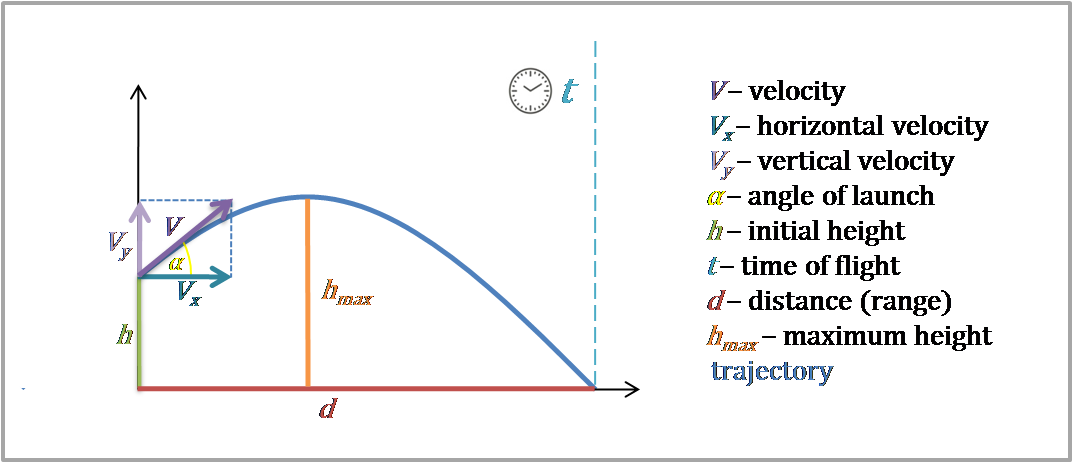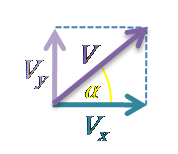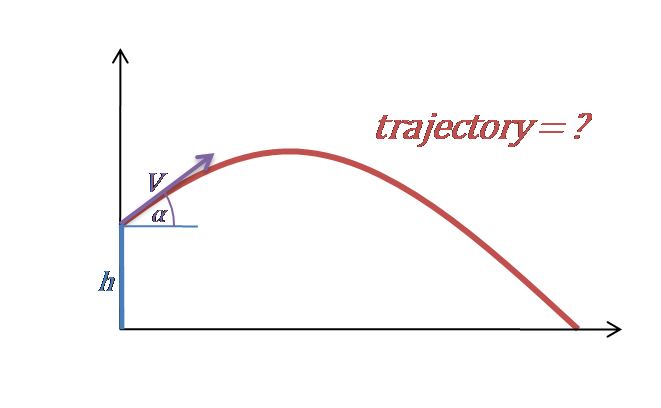# Trajectory Calculator

Created by Hanna Pamuła, PhD candidate
Reviewed by Bogna Szyk
Last updated: Mar 21, 2022

Use this trajectory calculator to find the flight path of a projectile. Type in three values: velocity, angle and initial height, and in no time you'll find the trajectory formula and its shape. Keep reading if you want to check the trajectory definition as well as a simple example of calculations.

## Trajectory definition

Trajectory, also called a flight path, is the path followed by a moving object under the action of gravity. Usually, the term is used when we're talking about projectiles or satellites (where the regularly repeating trajectory is called an orbit). In an object is thrown for short distances, then a parabola is a good approximation of trajectory shape.

Some examples of projectile motion are:

• ice hockey puck, baseball or golf ball in flight ⚾
• a bullet fired from a weapon 🔫
• a jet of water from a fountain ⛲
• object thrown from a table / plane / building / bridge ✈️

## Trajectory formula

Now as you know the trajectory definition, let's check how trajectory formula looks like.1. Let's start from equations of motion:
• x = Vₓ * t
• y = h + Vᵧ * t - g * t² / 2
1. As we know that three vectors - V₀, Vₓ and Vᵧ - form a right triangle, we can write that:• The horizontal velocity component Vₓ is equal to V₀ * cos(α).
• The vertical velocity component Vᵧ = V₀ * sin(α).
1. Then, we combine the equations of motion and velocity components into one formula:
• x = Vₓ * t => t = x / Vₓ
• y = h + Vᵧ * t - g * t² / 2
y = h + Vᵧ * (x / Vₓ) - g * (x / Vₓ)² / 2
• y = h + x * (V₀ * sin(α)) / (V₀ * cos(α)) - g * (x / V₀ * cos(α))² / 2

And as we know, sine over cosine is a definition of tangent. So, the final trajectory formula may be expressed as:

• y = h + x * tan(α) - g * x² / (2 * V₀² * cos²(α))

If you're interested in different aspects of projectile motion, check out our other dedicated tools to calculate:

## Trajectory calculator: how to use

Let's check out the trajectory of water from a water fountain:1. Type in the velocity. Assume that it's equal to 5 ft/s.
2. Enter the angle. For example, 60°.
3. Choose initial height. Let's pick 5 inches.
4. Here you are! Trajectory calculator displays the formula and the flight path!

The critical thing to notice is the possible difference in axes scaling, so the angle may not look like on the chart as it should. Remember that in all calculations, air resistance is neglected.

Hanna Pamuła, PhD candidate
Velocity
ft/s
Angle of launch
deg
Initial height
ft
Trajectory formula:
y = h + xtan(α) - gx²/2V₀²cos²(α)People also viewed…

### Free space path loss

The free space path loss calculator allows you to predict the strength of a radio frequency signal emitted by an antenna at any given distance.

### Lost socks

Socks Loss Index estimates the chance of losing a sock in the laundry.

### Manometer

This manometer calculator will determine pressures at specific points in any common manometer. Manometers are simple to use and knowing how to use them is a fantastic way to learn more about fluid statics.

### Sleep

The sleep calculator can help you determine when you should go to bed to wake up happy and refreshed.